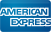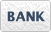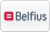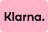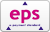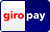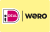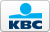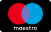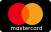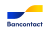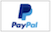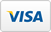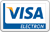EUR

# Rounding over bits for woodworking

Rounding over bits for woodworking

-14%
Titman
rounding over cutter HW R=1,6 S=8
rounding over cutter HW Z=2 D=19,2 R=1,6 L=8 S=8
€76,42 €76,42 €66,00 €66,00

-14%
Titman
Rounding over bit HW R=1,6 S=6
Rounding over bit HW Z=2 D=19,2 R=1,6 L=8 S=6
€76,42 €76,42 €66,00 €66,00

-12%
Titman
Titman Rounding over bit R=1 S=8
Titman Rounding over bit R=1 S=8
€76,42 €76,42 €67,00 €67,00

-11%
Titman
Rounding over bit HW R=2 S=8
Rounding over bit HW Z=2 D=20 R=2 L=10 S=8
€76,28 €76,28 €68,00 €68,00

-11%
Titman
Titman Rounding over bits R=2 S=6
Titman Rounding over bits R=2 S=6
€76,28 €76,28 €68,00 €68,00

-10%
Titman
Rounding over cutter HW R=3 S=8
Rounding over cutter HW Z=2 D=22 R=3 L=10 S=8
€76,28 €76,28 €69,00 €69,00

-10%
Titman
Titman Rounding over bits R=3 S=6
Titman Rounding over bits R=3 S=6
€76,28 €76,28 €69,00 €69,00

-8%
Titman
Titman Rounding over bits R=4 S=8
Titman Rounding over bits R=4 S=8
€76,28 €76,28 €70,00 €70,00

-8%
Titman
Rounding over bit HW R=4 S=6
Rounding over bit HW Z=2 D=24 R=4 L=10 S=6
€76,28 €76,28 €70,00 €70,00

Titman
Rounding over cutter with guide pin shank 8 mm
Rounding over cutter with guide pin shank 8 mm
€70,22 €70,22

-10%
Titman
Titman Rounding over bit R=6 S=8
Titman Rounding over bit R=6
€78,82 €78,82 €71,00 €71,00

Titman
Rounding over cutter
Rounding over cutter
€71,21 €71,21

-9%
Titman
Titman rounding over cutter HW R=6.3 S=8
Titman rounding over cutter HW Z=2 D=28.7 R=6.3 L=12 S=8
€78,82 €78,82 €72,00 €72,00

-18%
PCD rounding over bit R2 S=8 mm
Diamond roundingover bit radius 2 mm Shank 8 mm
€90,00 €90,00 €74,00 €74,00

-11%
Titman
Miniature rounding over cutter R=2 S=8
Titman rounding over cutter R=2 S=8
€83,03 €83,03 €74,00 €74,00

-8%
Titman
Titman rounding over bit HW R=8 S=8
Titman rounding over bit HW Z=2 D=32 R=8 L=14 S=8
€80,63 €80,63 €74,00 €74,00

-8%
Titman
Titman rounding over bit HW R=8 S=6
Titman rounding over bit HW Z=2 D=32 R=8 L=14 S=6
€80,63 €80,63 €74,00 €74,00

-17%
PCD rounding over bit R1,6 S=8 mm
Diamond roundingover bit radius 1.6 mm Shank 8 mm
€90,00 €90,00 €75,00 €75,00

-11%
Titman
Titman rounding over bit HW R=6.3 S=6
Titman rounding over bit HW Z=2 D=28.7 R=6.3 L=12 S=6
€84,18 €84,18 €75,00 €75,00

-9%
Titman
Titman Rounding over bits R=5 S=8
Titman Rounding over bits R=5
€82,67 €82,67 €75,00 €75,00

Showing 1 - 24 of 53Rounding over bits for woodworking

##### Price
Min Max